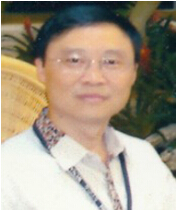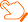### 近期论文查看导师最新文章 （温馨提示：请注意重名现象，建议点开原文通过作者单位确认）

Coupled second order evolution equations with fading memory: optimal energy decay rate, J. Differential Equations 257 (2014), 1501-1528. (with K. P. Jin, T. J. Xiao) （美，SCI） A fixed point theorem for cyclic mappings in complete metric spaces, J. Nonlinear Convex Anal. 15 (2014), 1–6. (with R. P. Agarwal, J. Zhang) （日，SCI） Refinements of the Heinz operator inequalities, Linear Multilinear Algebra, to appear. (with G. H. Shi ) （美，SCI） Common fixed points and invariant approximations for noncommuting contraction mappings in strongly convex metric spaces, J. Nonlinear Convex Anal., to appear. (with S. Chandok, D. O‘Regan) （日，SCI） Comparison of differences among power means $Q_{r,\alpha}(a,b,\mathbf{x})s$, J. Math. Inequal., to appear. (with G. H. Shi ) （SCI） Weighted pseudo almost automorphic functions and WPAA solutions to semilinear evolution equations, J. Math. Anal. Appl. 409 (2014), 409–427. (with H. S. Ding,T. J. Xiao) （美，SCI） Coupled second order semilinear evolution equations indirectly damped via memory effects, J. Differential Equations 254 (2013), 2128–2157. (with T. J. Xiao) （美，SCI） Integral solution of a class of nonlinear integral equations, Appl. Math. Comput. 219 (2013) 4950-4957. (with S. H.Yan, R. P. Agarwal, T. W. Huang) （美，SCI） Weighted pseudo almost periodic functions and applications to evolution equations with delay, Appl. Math. Comput. 219 (2013), 8949–8958. (with H. S. Ding,T. J. Xiao) （美，SCI） On perturbation of convoluted $C$-regularized operator families, J. Funct. Spaces Appl. 2013 (2013), Article ID 579326, 8 pages. (with F. Li, T. J. Xiao, J. Zhang)（美，SCI） A note on existence of solutions to the Cauchy problem for a class of nonlinear evolution equations, Nonlinear Studies, 20 (2013), 237-247. (with F. Li, T. J. Xiao, J. Zhang) Stability for a class of differential equations with nonconstant delay, J. Funct. Spaces Appl. 2013 (2013), Article ID 159435, 7 pages. J. Funct. Spaces Appl. (with T. T. Lu, Y. Xu) （美，SCI） Nonautonomous semilinear second order evolution equations with generalized Wentzell boundary conditions, J. Differential Equations 252 (2012), 3953-3971. （with T. J. Xiao）（美，SCI） A problem concerning Yamabe-type operators of negative admissible metrics, J. Appl. Math., 2012 (2012), Article ID 930868, 11 pages. （with H. Zhu）（美，SCI） On the existence of mild solutions to the Cauchy problem for a class of fractional evolution equations, Adv. Diff. Equ., 2012 (2012), 40. （with S. H. Yan, F. Li, T. W. Huang）（美，SCI） A nonlocal Cauchy problem for fractional integrodifferential equations, J. Appl. Math., 2012 (2012), Article ID 901942, 18 pages. （with F. Li, T. T. Lu, H. Zhu）（美，SCI） Nonlinear functions and the norm of the propagators of operational equations, Commun. Nonlinear Sci. Numer. Simul., (2012), （with Y. Lin, T. J. Xiao, T. W. Huang）（美，SCI） Weighted pseudo almost-periodic functions and applications to semilinear evolution equations, Abstr. Appl. Anal.，2012 (2012), Article ID 179525, 15 pages. （with J. Zhang, T. J. Xiao）（美，SCI） Existence of mild solutions for fractional integrodifferential equations of Sobolev type with nonlocal conditions, J. Math. Anal. Appl., 391 (2012), 510-525.（with F. Li, H. K. Xu）（美，SCI） Pseudo almost periodic solutions to integro-differential equations of heat conduction in materials with memory, Nonlinear Analysis: Real World Applications, 13 (2012).（with H. S. Ding, T. J. Xiao）（美，SCI）

down
wechat